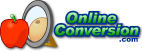## Welcome to OnlineConversion.com

How do I convert Square (something) to Cubic (something)?

One of the most common questions I am asked, is how to convert square feet to cubic feet. My answer has always been, "You can't." Since cubic feet has an extra dimension, you could fit an unlimited number of square feet into cubic feet.

But then I started thinking about why someone would want to make such a conversion, and I guess it is possible in the real world, and decided to put the conversion up here. Though scientists might scoff and say that I'm not really converting square feet to cubic feet, I'm just adding the third dimension that is needed for cubic feet.

First off, why would someone need to convert square feet to cubic feet? As an example, lets say you have a 12 square foot garden, and you want to buy topsoil for it. Since topsoil is sold by the cubic foot, how many cubic feet of topsoil do you need for a 12 square foot garden?  I will use this for my example.

The formula for Square feet is:
length × width

The formula for Cubic feet is:
length × width × height

Since we know the Square feet of the garden, we now have the conversion to Cubic feet:
(cubic feet) = (square feet) × (height in feet)

So.... If you wanted the topsoil to be 3 inches thick, you would need:
12 × 0.25 = 3
You would need 3 cubic feet of topsoil.

Notice that I convert 3 inches to 0.25 feet before using the formula. All the measurements must be in the same units for it to work. And it will work for anything. Meters, feet, inches, just as long as all are the same.

Once again, here is the formula:
(cubic feet) = (square feet) × (height in feet)

Or for meters:
(cubic meters) = (square meters) × (height in meters)### BookMark Us

It may come in handy.

Check out our Conversion Software for Windows.Can't find something?
Try searching.

Are you bored?
Try the Fun Stuff.